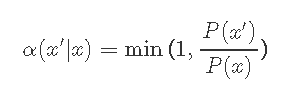# 詳解R語言MCMC:Metropolis-Hastings采樣用於回歸的貝葉斯估計

MCMC是從復雜概率模型中采樣的通用技術。

1. 蒙特卡洛
2. 馬爾可夫鏈
3. Metropolis-Hastings算法

## 問題1.1直接模擬

1.2逆CDF

1.3拒絕/接受抽樣

## 馬爾可夫鏈馬爾可夫鏈的收斂性意味著它具有平穩分佈π。馬爾可夫鏈的統計分佈是平穩的,那麼它意味著分佈不會隨著時間的推移而改變。

## Metropolis算法

對於一個Markov鏈是平穩。基本上表示## Metropolis-Hastings算法

A的選擇遵循以下邏輯。3.接受根據α（x’| x）的狀態。如果不接受，則不會進行轉移，因此無需更新任何內容。否則，轉移為x’；

4.轉移到2，直到生成T狀態；

5.保存狀態x，執行2。

## 屬性

Metropolis-Hastings算法的一個有趣特性是它 僅取決於比率## Metropolis采樣## MH可視化

```set.seed(123)

for (i in 2:n) {
can <- rnorm(1, mu, sig)
aprob <- min(1, (dgamma(can, a, b)/dgamma(x,
a, b))/(dnorm(can, mu, sig)/dnorm(x,
mu, sig)))
u <- runif(1)
if (u < aprob)
x <- can
vec[i] <- x```

## 畫圖

```nrep<- 54000
burnin<- 4000
shape<- 2.5
rate<-2.6```

```vec=vec[-(1:burnin)]
#vec=vec[burnin:length(vec)]```
```par(mfrow=c(2,1)) # 更改主框架，在一幀中有多少個圖形
plot(ts(vec), xlab="Chain", ylab="Draws")
abline(h = mean(vec), lwd="2", col="red" )``````Min. 1st Qu.  Median   Mean 3rd Qu.   Max.
0.007013 0.435600 0.724800 0.843300 1.133000 3.149000```
`var(vec[-(1:burnin)])`
` 0.2976507`

## 初始值

```  x <- 3*a/b
vec <- x```

## 選擇方案

Metropolis-Hastings采樣用於貝葉斯估計回歸模型。DGP和圖

```# 創建獨立的x值，大約為零
x <- (-(Size-1)/2):((Size-1)/2)
# 根據ax + b + N（0，sd）創建相關值
y <- trueA * x + trueB + rnorm(n=Size,mean=0,sd=trueSd)``````pred = a*x + b
singlelikelihoods = dnorm(y, mean = pred, sd = sd, log = T)
sumll = sum(singlelikelihoods)```

```# 示例：繪制斜率a的似然曲線
plot (seq(3, 7, by=.05), slopelikelihoods , type="l")```## 先驗分佈

```# 先驗分佈

# 更改優先級，log為True，因此這些均為log
density/likelihood
aprior = dunif(a, min=0, max=10, log = T)
bprior = dnorm(b, sd = 2, log = T)
sdprior = dunif(sd, min=0, max=30, log = T)```

## 後驗

```posterior <- function(param){
return (likelihood(param) + prior(param))
}```

## Metropolis算法

1. 上面定義的後驗。
2. 從隨機參數值開始
3. 根據某個候選函數的概率密度，選擇一個接近舊值的新參數值
4. 以概率p（new）/ p（old）跳到這個新點，其中p是目標函數，並且p> 1也意味著跳躍
5. 請註意，我們有一個 對稱的跳躍/候選分佈 q（x’| x）。```######## Metropolis 算法 ################

for (i in 1:iterations){

probab = exp(posterior(proposal) - posterior(chain[i,]))
if (runif(1) < probab){
chain[i+1,] = proposal
}else{
chain[i+1,] = chain[i,]
}```

## 實施

（e）輸出接受的值，並解釋。

```chain = metrMCMC(startvalue, 5500)

burnIn = 5000
accep = 1-mean(duplicated(chain[-(1:burnIn),]))```

`startvalue = c(4,0,10)`

## 小結

``` V1       V2        V3
Min.  :4.068  Min.  :-6.7072  Min.  : 6.787
1st Qu.:4.913  1st Qu.:-2.6973  1st Qu.: 9.323
Median :5.052  Median :-1.7551  Median :10.178
Mean  :5.052  Mean  :-1.7377  Mean  :10.385
3rd Qu.:5.193  3rd Qu.:-0.8134  3rd Qu.:11.166
Max.  :5.989  Max.  : 4.8425  Max.  :19.223```
```#比較:
summary(lm(y~x))```
```Call:
lm(formula = y ~ x)

Residuals:
Min   1Q Median   3Q   Max
-22.259 -6.032 -1.718  6.955 19.892

Coefficients:
Estimate Std. Error t value Pr(>|t|)
(Intercept) -3.1756   1.7566 -1.808  0.081 .
x       5.0469   0.1964 25.697  <2e-16 ***
---
Signif. codes: 0 ?**?0.001 ?*?0.01 ??0.05 ??0.1 ??1

Residual standard error: 9.78 on 29 degrees of freedom
Multiple R-squared: 0.9579,  Adjusted R-squared: 0.9565
F-statistic: 660.4 on 1 and 29 DF, p-value: < 2.2e-16```
`summary(lm(y~x))\$sigma`
` 9.780494`
`coefficients(lm(y~x))`
```(Intercept)
-3.175555```
`coefficients(lm(y~x))`
```   x
5.046873```

```### 總結: #######################

par(mfrow = c(2,3))
hist(chain[-(1:burnIn),1],prob=TRUE,nclass=30,col="109"
abline(v = mean(chain[-(1:burnIn),1]), lwd="2")```